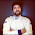### Getting LED Indicators to function correctly.

Because we were running a smaller battery in size the amount of current it supplied was lower than the bulky stock battery.  Therefore we decided to replace our globed indicators and taillights with the LED variety.

I bought a set of LED indicators and also sourced a LED indicator flasher relay which "promised" to fix the fast flashing/no flashing which occurs when swapping over to LEDS.

This was not the case for some reason the LEDS were still not drawing enough current to make them flash.  So after a bit of research I found out that you can buy bike specific resistors to fit in sequence with your indicators to replicate the same current draw as what the existing indicators did.  I still wasn't convinced that this would be the correct solution as I thought that the LED flasher relay would have solved it, then I decided to do my own calculations to work out what resistance was required and head to JayCar to pick up my own resistors.

Using Ohm's Law, which I hadn't used since high school I was able to find out the resistance needed for the LEDS to draw the same current as the bulbs.Soldered inline resistors
It felt like I was in high school again minus the undercut and the grunge music in the background.  As taken from the the12Volt.com

"Ohm's Law defines the relationships between (P) power, (E) voltage, (I) current, and (R) resistance. One ohm is the resistance value through which one volt will maintain a current of one ampere. "

V = I x R

So in a 12V system the XS250 indicators were rated at 27Watts

Now to work out what's required for the LEDS we subtract the LEDS electrical power from that of the bulbs

27 - 1 (1 Watt Rating)

Power is calculated at Current x Voltage

P = I x V

so 26 = I x 12V

so the current for each side would be 2.2Amps

Finally we get to the resistance, and using OHM's we get
V = I x R
12V = 2.2 x R

R = 12/2.2

R = 5.5Ohms

So I went out and bought a couple of types a 5.6 and a 8.2Ohm resistor one for each side.  We soldered them inline and the 5.6 worked fine however because it would be drawing much more current than than the 8.2Ohm I decided to use that instead.

Now they work a treat.